# NCERT Solutions Class 12

• ##### NCERT Solutions-Biology
No items in this section

## NCERT Solutions Class 12

### Exercise 4.1

Evaluate the following determinants in Exercise 1 and 2.

1.Ans.==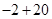= 18

2. (i)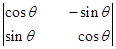(ii)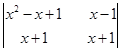Ans. (i)=== 1

(ii)=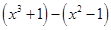=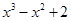### 3. If A =then show that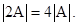Ans. Given: A =then 2A =L.H.S. =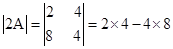=R.H.S. ==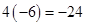Since L.H.S. = R.H.S.

Hence, proved.

4. If A =then show that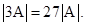Ans. Given: A =then 3A ==L.H.S.   =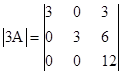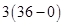= 3 x 36 = 108

R.H.S.   == 27 [1 (4 – 0)] = 27 x 4 = 108

Since   L.H.S. = R.H.S.

Hence, proved.

5. Evaluate the determinants:

(i)(ii)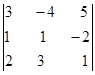(iii)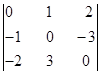(iv)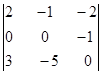Ans. Evaluate the determinants:

(i) Given:Expanding along first row,=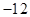(ii) Given:Expanding along first row,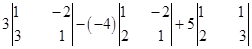= 21 + 20 + 5 = 46

(iii) Given:Expanding along first row,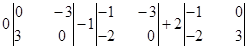= 0 + 6 – 6 = 0

(iv) Given:Expanding along first row,= 5

6. If A =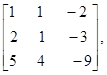find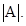Ans. Given:     A =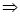=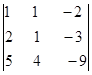Expanding along first row,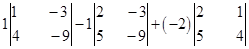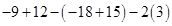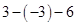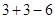= 0

7. Find the value ofif:

(i)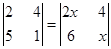(ii)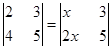Ans. (i) Given: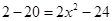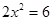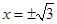(ii)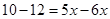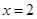8. Ifthen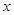is equal to:

(A) 6

(B)(C)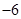(D) 0

Ans. Given: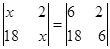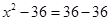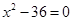Therefore, option (B) is correct.